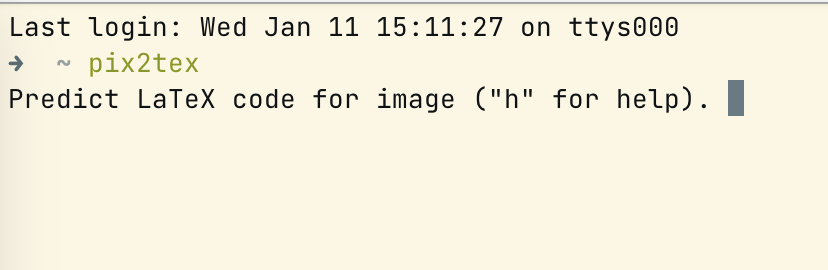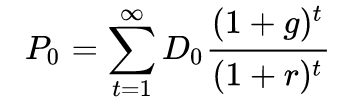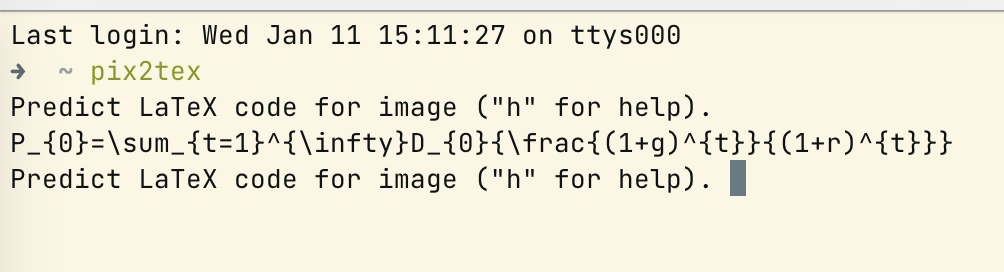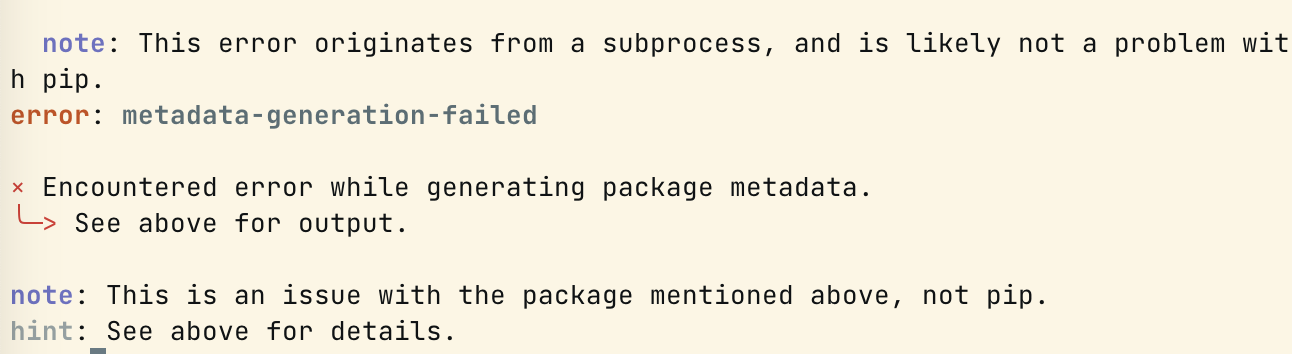# Mathpix Snipping alternative for latex math equation

I wrote this post for M-series chip since some dependencies cannot run on the ARM platform.

This post introduces an alternative tool for LaTex code. I used Mathpix for a long time. However, it only provides 10 snips a month if you do not have a subscription.

Then I find LaTex-OCR project from GitHub.You can easily follow the official doc to install and use this project. The precondition is you have installed Python on your system.

You can install any native version ($$\geq$$ 3.7) of Python via Homebrew or asdf.

Once you installed Python, you can install the project by pip

The system will install pix2tex with dependencies.

## Run with terminal

Then open the terminal and run the project with code pix2tex.Then take a screenshot of the wanted equation and copy it. Then press enter to render the code. For example, I want the DDM equation:The project will render code as follow:$P_{0}=\sum_{t=1}^{\infty}D_{0}{\frac{(1+g)^{t}}{(1+r)^{t}}}$

## Run with GUI

The project is built on PyQt5. However PyQt5 cannot run on M1-series natively.

If you want to install PyQt5 via arm64, the system will return an error:You should run python and terminal via Rosetta x86. I posted an instruction on how to install x86 python here. You can use the same method to install python and run pix2tex with GUI.

### install x86 Python

Just follow the post and finish the setting.

### Install pix2tex[gui]

Run the following code to install:

Open GUI with code:

The system will start the project like this:You can snip the equation. However, the performance is unstable. The GUI constantly crash.

The problem may be fixed in the next version after updating to PyQt6.

Mathpix Snipping alternative for latex math equation
https://www.pengjiaxin.com/2023/01/11/latexocr/
Author
Peng Jiaxin
Posted on
January 11, 2023